## Characteristics of DC Motors | Characteristics of Shunt Motor:

The power of the dc motor lies in its versatility and ease with which a variety of speed-torque characteristics can be obtained, and the wide range of speed control which is possible without the need of elaborate control schemes while a high level of operating efficiency is maintained. In a de generator the speed is fixed by the prime-mover and remains nearly constant throughout the operating part of the Characteristics of DC Motors, while the field conditions are adjusted to yield the desired terminal voltage at a given load. In a motor, on the other hand, the need is to match the speed-torque Characteristics of DC Motors of the load and run the load at a specified speed or speeds by adjustment of the field and by adjustment of the armature voltage in case speed control over a wide range is desired.

The fundamental emf and torque relationships of Equations are reproduced below: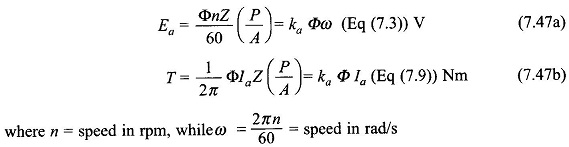In motoring operation it is convenient to express these relationships in the form of armature speed and torque, i.e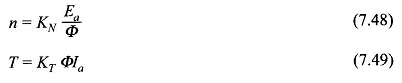It is observed that Ea is governed by the armature circuit equation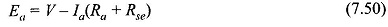and the flux/pole Φ is determined by the equivalent excitation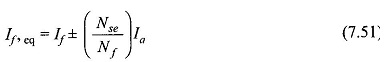and the magnetization characteristic of the machine with the proviso that the armature reaction will cause a reduction in flux/pole.

DC motors are of three types according to how these are excited. In a motor the shunt excitation is not dependent on the armature induced emf and is independently governed by the supply voltage. In this regard shunt excitation of motor behaves more like separate excitation and not like self-excitation as in a shunt-excited generator.

### Characteristics of Shunt Motor:

Figure 7.41 shows the connection diagram of a shunt motor. Its operation with a fixed terminal voltage and fixed field resistance, i.e. with constant shunt field current will be now considered.

On the armature circuit Ea = V– laRa                                                                      (7.52)

which upon substitution in Eq. (7.48) gives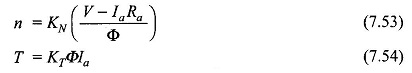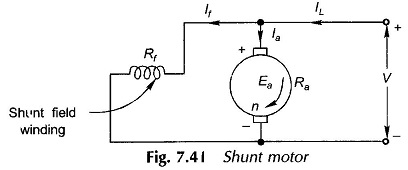Equations (7.53) and (7.54) yield the variation of motor speed with armature current and with torque as plotted in Figs. 7.42(a) and (b). These characteristics can also be plotted with respect to line current by merely adding the field current (IL = Ia + If).

It follows from Eq. (7.53) that speed will fall off due to armature resistance drop IaRa but this is countered by a decrease in Φ caused by the armature reaction. Since IaRa effect predominates, the speed falls somewhat with load as shown in Fig. 7.42(a). As per Eq. (7.54), but for demagnetizing effect of armature current, the T-Ia characteristic would be straight line slightly sloping downwards. The actual torque characteristic is somewhat higher and bends inwards due to reduction in flux/pole as shown in Fig. 7.42(a).

Eliminating Ia between Eqs (7.53) and (7.54) the speed-torque characteristic is obtained aswhich would be a straight line but for reduction in Φ caused by the armature reaction. The actual characteristic is plotted in Fig. 7.42(b). The speed drops off from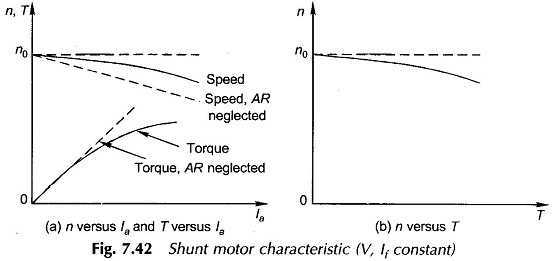no-load to full-load by a few per cent; in fact the speed remains substantially constant. Such a characteristic is known as the “shunt” characteristic. An important class of ac machine, called the induction motor, also possesses a similar (n-T) characteristic.

Scroll to Top